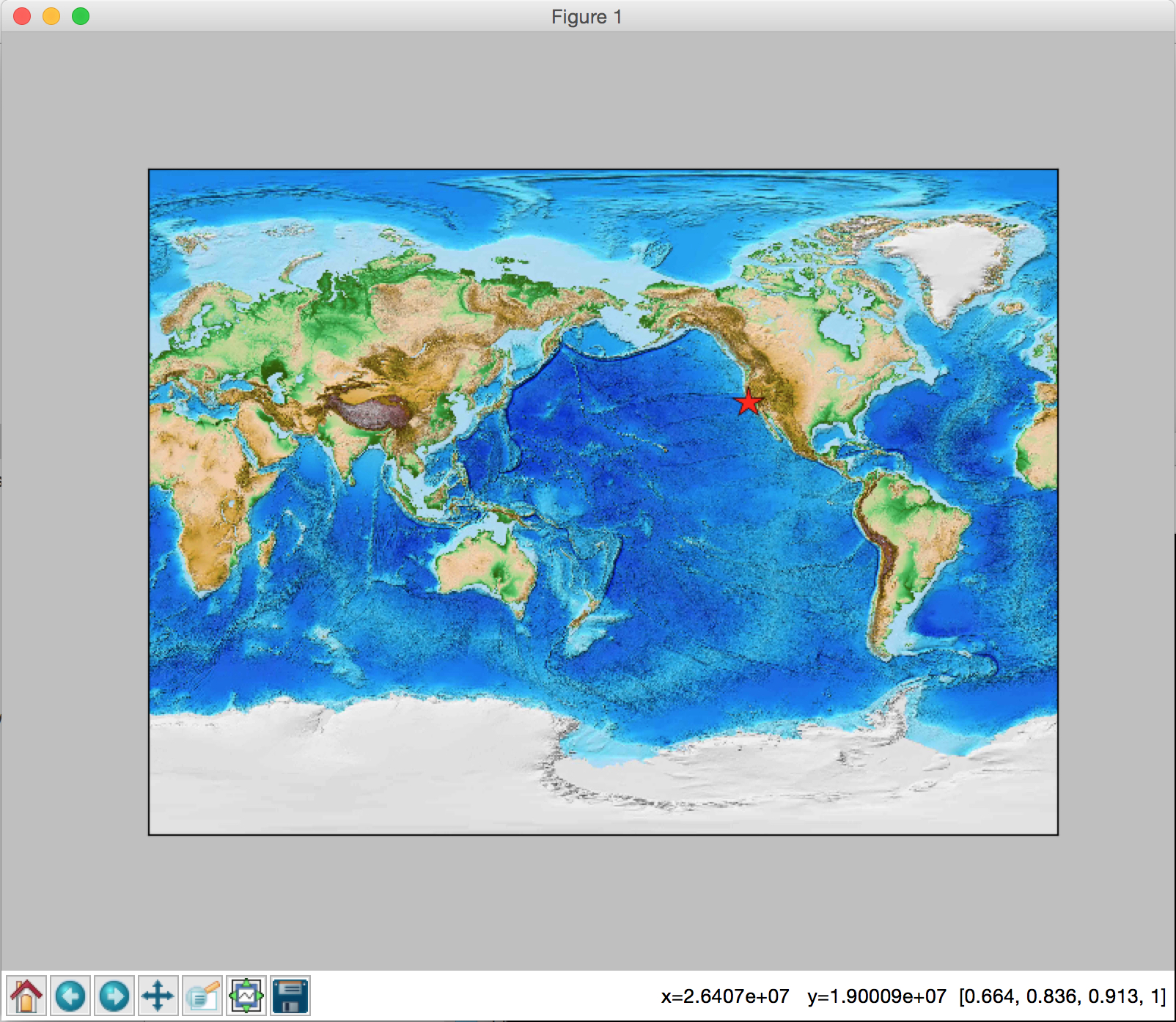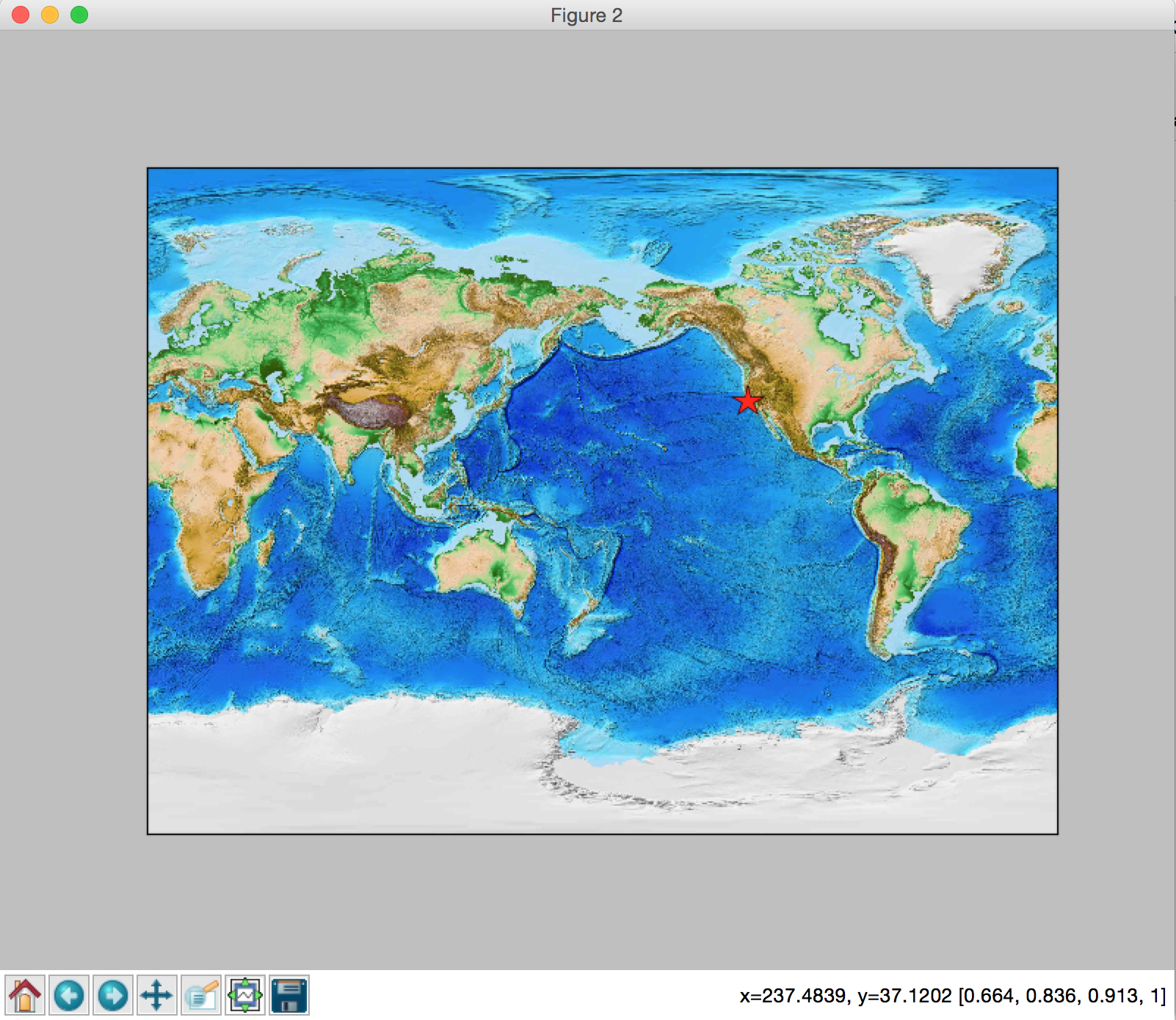## Sunday, December 18, 2016

### Python: Display Latitude/Longitude on Basemap when moving cursor

When we make a map using Basemap, sometimes we want to get the latitude and longitude directly on the map when we move the cursor. But we find that the numbers showing on the right bottom corner of the figure are not the latitude and longitude. What are the numbers showing here? They are the values converted from the longitude and latitude according to certain projection (in meters). Therefore, if we want to show latitude and longitude on the figure, we need convert them back. You can find the code at Qingkai's Github
Let's see an example, where I will plot my location on the map. We can see the numbers are x = 2.64707e+07, y = 1.90009e+07.
from mpl_toolkits.basemap import Basemap
import matplotlib.pyplot as plt
plt.figure(figsize = (10,8))

# miller projection
map = Basemap(projection='mill',lon_0=180)

# let's have a nice background
map.etopo()

# let's add Berkeley (my location) on the map
my_lat = 37.8716
my_lon = 237.7273

# convert lat/lon to x/y map projection coordinates (in meters)
x,y = map(my_lon, my_lat)

# plot the star
map.plot(x, y, 'r*', markersize = 20, zorder = 10)

plt.show()## Let's change the x, y to latitude and longitude

# we define a helper function to convert x/y value back to lat/lon
# all we need do is to do a reverse operation on the x/y value
def format_coord(x, y):
return 'x=%.4f, y=%.4f'%(map(x, y, inverse = True))
plt.figure(figsize = (10,8))

# miller projection
map = Basemap(projection='mill',lon_0=180)

# let's have a nice background
map.etopo()

# let's add Berkeley (my location) on the map
my_lat = 37.8716
my_lon = 237.7273

# convert lat/lon to x/y map projection coordinates (in meters)
x,y = map(my_lon, my_lat)

# plot the star
map.plot(x, y, 'r*', markersize = 20, zorder = 10)

ax = plt.gca()
ax.format_coord = format_coord

plt.show()Now we can see that we converted the number back to x = Longitude, and y = Latitude. This way we can easily get the latitude and longitude we want.